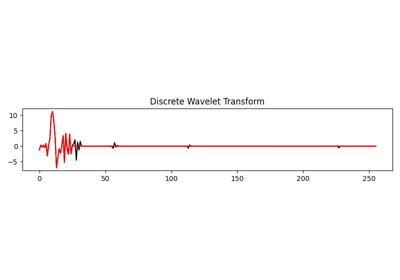# pylops.signalprocessing.DWT#

class pylops.signalprocessing.DWT(dims, axis=-1, wavelet='haar', level=1, dtype='float64', name='D')[source]#

One dimensional Wavelet operator.

Apply 1D-Wavelet Transform along an axis of a multi-dimensional array of size dims.

Note that the Wavelet operator is an overload of the pywt implementation of the wavelet transform. Refer to https://pywavelets.readthedocs.io for a detailed description of the input parameters.

Parameters
dims

Number of samples for each dimension

axisint, optional

New in version 2.0.0.

Axis along which DWT is applied

waveletstr, optional

Name of wavelet type. Use pywt.wavelist(kind='discrete') for a list of available wavelets.

levelint, optional

Number of scaling levels (must be >=0).

dtypestr, optional

Type of elements in input array.

namestr, optional

New in version 2.0.0.

Name of operator (to be used by pylops.utils.describe.describe)

Raises
ModuleNotFoundError

If pywt is not installed

ValueError

If wavelet does not belong to pywt.families

Notes

The Wavelet operator applies the multilevel Discrete Wavelet Transform (DWT) in forward mode and the multilevel Inverse Discrete Wavelet Transform (IDWT) in adjoint mode.

Wavelet transforms can be used to compress signals and present a key advantage over Fourier transforms in that they captures both frequency and location information in time. Consider using this operator as sparsifying transform when using L1 solvers.

Attributes
shapetuple

Operator shape

explicitbool

Operator contains a matrix that can be solved explicitly (True) or not (False)

Methods

 __init__(dims[, axis, wavelet, level, ...]) adjoint() apply_columns(cols) Apply subset of columns of operator cond([uselobpcg]) Condition number of linear operator. conj() Complex conjugate operator div(y[, niter, densesolver]) Solve the linear problem $$\mathbf{y}=\mathbf{A}\mathbf{x}$$. dot(x) Matrix-matrix or matrix-vector multiplication. eigs([neigs, symmetric, niter, uselobpcg]) Most significant eigenvalues of linear operator. matmat(X) Matrix-matrix multiplication. matvec(x) Matrix-vector multiplication. reset_count() Reset counters rmatmat(X) Matrix-matrix multiplication. rmatvec(x) Adjoint matrix-vector multiplication. todense([backend]) Return dense matrix. toimag([forw, adj]) Imag operator toreal([forw, adj]) Real operator tosparse() Return sparse matrix. trace([neval, method, backend]) Trace of linear operator. transpose()

## Examples using pylops.signalprocessing.DWT#Wavelet transform

Wavelet transform